Algebra 1 : Systems of Inequalities

Example Questions

1 2 5 6 7 8 9 10 11 13 Next →

Example Question #36 : How To Find The Solution To An Inequality With Division

Solve the following inequality: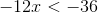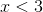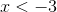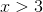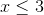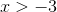Explanation:

Divide by negative twelve on both sides of the inequality.

Dividing by a negative value requires changing the direction of the sign.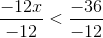Simplify both sides.

The answer is:Example Question #37 : How To Find The Solution To An Inequality With Division

Solve the inequality: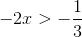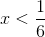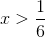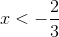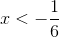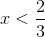Explanation:

Divide both sides by negative two.  We will need to switch the direction of the sign.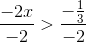Simplify both sides and switch the sign.  Rewrite the complex fraction such that dividing by a negative two is similar to multiplying by negative half.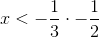Multiply the fractions on the right.

The answer is:Example Question #38 : How To Find The Solution To An Inequality With Division

Solve the inequality: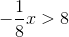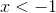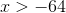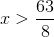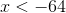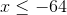Explanation:

Dividing by negative one-eighth is similar to multiplying by negative eight on both sides.  Since we are dividing by a negative number, the sign will switch.Simplify both sides.

The answer is:Dividing by negative one-eighth is similar to multiplying by negative eight on both sides.  Since we are dividing by a negative number, the sign will switch.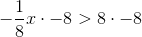Simplify both sides.

The answer is:1 2 5 6 7 8 9 10 11 13 Next →